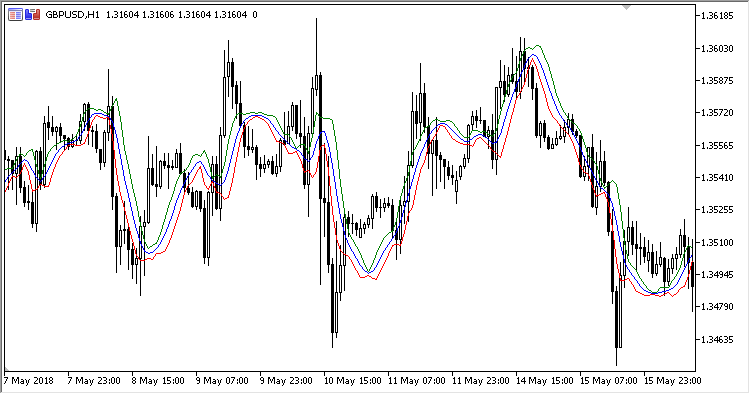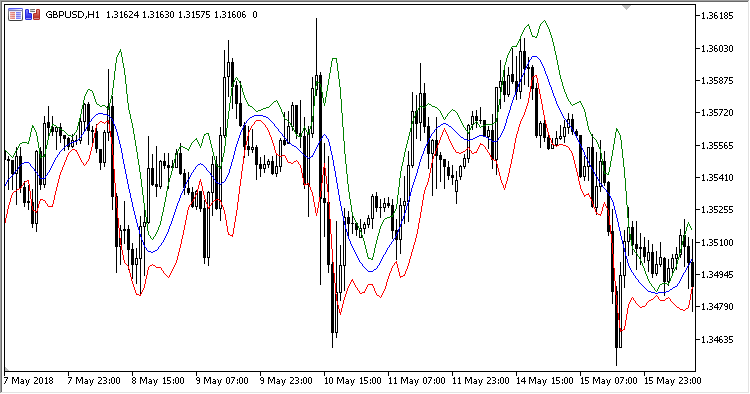192

2018.11.08 09:42

• Regression period - 线性回归周期数
• Smoothing period （平滑周期数）
• Multiplier - 标准差比例
• Applied price

```Central = SMA(LReg,Smoothing period)
Top    = Central + Multiplier * Deviation
Bottom = Central - Multiplier * Deviation
```

```Deviation = StdDev(LReg, Smoothing period)
LReg - 在 'Applied price' 使用 'Regression period' 计算周期数的线性回归
```Volatility_StopVolatility_Stop_OscillatorSSSARMurreys_Math_Oscillator

Murreys Math 振荡指标